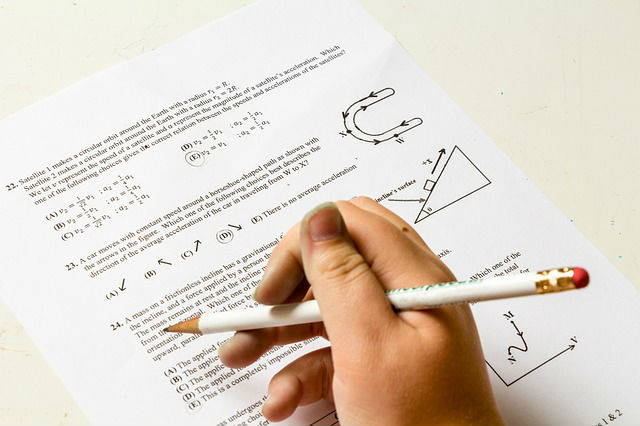# Solution to problems – physics class 9 – Set 1 Q42, Q43, Q44, Q45, Q46

Last updated on January 2nd, 2020 at 08:31 am

## Problem Statement

42) A force of 20 N acts upon a body whose weight is 9.8 N. What is the mass of the body and how much is its acceleration ? (g = 9.8 m/ s^2).

Solution (Q42)

Weight = W = 9.8 N,  g = 9.8 m s-2

Weight (W) = mass * acceleration due to gravity
W= mg
Mass of the body(m) = W / g = 9.8 /9.8 = 1 kg

Force = mass x acceleration
so, Acceleration = force /mass = 20 / 1 = 20 m/s2

43) A stone resting on the ground has a gravitational force of 20 N acting on it. What is the weight of the stone ?What is its mass ? ( g = 10 m/s^2).

Solution (Q43)

Weight = gravitational force = 20 N
Mass = weight / g = 20/10 = 2 kg

44)
(a) An object has mass of 20 kg on earth.
What will be its (i) mass, and (ii) weight, on the moon?(g on moon = 1.6 m/s^2).
(b) Calculate the acceleration due to gravity on the surface of a satellite having a mass of 7.4 × 10^22 kg and a radius of 1.74 × 10^6 m (G = 6.7 × 10 -11 Nm^2/kg^2).

Solution (Q44)

(a) i) Mass on the moon = 20 Kg
ii) Weight on the moon = m gmoon= 20 * 1.6 = 32 N
(b) say, gsat  is the acceleration due to gravity on the surface of the satellite.
Then, gsat = GM/r2 = (6.7 × 10 -11 x 7.4 × 1022) / (1.74 × 106)2 = 1.63 m/s2

[We get the eqn. of gsat in this way:
weight of an object of mass m on the satellite is mgsat
The gravitational force on that mass m due the satellite of M is GMm/r2,
where r is the radius of the satellite.
weight of the object on satellite = gravitational force on the object due to the satellite
mgsat = GMm/r2
gsat = GM/r2 ]

See also  Solution to problems - class 9 physics - Set 1 Q 54

45) The mass of a planet is 6 × 10^24 kg and its diameter is 12.8 × 10^3 km. If the value of gravitational constant be 6.7 × 10 -11 Nm2/kg2, calculate the value of acceleration due to gravity on the surface of the planet.

Solution (Q45)

Diameter of the planet= 12.8 × 103 km = 12.8 x 106 m
Radius = 6.4 x 106 m

gplanet = GM/r2 = (6.7× 10 -11 x 6× 1024) / (6.4 × 106)2

= 9.8 m/s2

46) The values of g at six distances A, B, C, D, E and F from the surface of the earth are found to be 3.08 m/s^2, 9.23 m/s^2, 0.57 m/s^2, 7.34 m/s^2, 0.30 m/s^2 and 1.49 m/s^2, respectively.

(a) Arrange these values of g according to the increasing distances from the surface of the earth (keeping the value of g nearest to the surface of the earth first)

(b) If the value of distance F be 10000 km from the surface of the earth(hint: check if possible or not), state whether this distance is deep inside the earth or high up in the sky.

Solution (Q46)

(a) As we know, as height increases from
the surface of earth the value of g decreases.
The values of g according to the
increasing distances from the surface of the earth (keeping the value of g nearest to the surface of the earth first) are 9.23, 7.34, 3.08, 1.49,0.57,0.3 (all with units m/s2

See also  Solution to problems - class 9 physics - Set 1 Q 58

(b) The diameter of earth is 6400 km only. So this 10000 km distance can’t be deep inside the earth. This must be high up in the sky.

Go back to the 101 problems set (class 9)